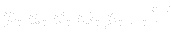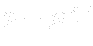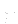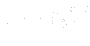This is an automatically generated documentation by LaTeX2HTML utility. In case of any issue, please, contact us at info@cfdsupport.com.

## Equation of state

The number of mentioned governing equations is five, but gives seven unknowns:. This is why the system has to be extended by the state equation (for ideal gas):(21.13)

Wheredenotes the spacific gas constant. Using the state equation and the thermodynamics laws, the internal energy can be expressed by the following equation21.5:(21.14)